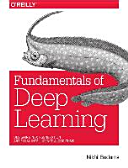### Deep Learning

#### Course Description

Deep learning is a branch of machine learning based on representation of data with complex representations at a high level of abstraction. These representations are achieved by a sequence of trained non-linear transformations. Deep learning methods have been successfully applied in many important artificial intelligence fields such as computer vision, natural language processing, speech and audio understanding as well as in bioinformatics. This course introduces the most important deep discriminative and generative models with a special focus on practical implementations. Part one introduces key elements of classical feed-forward neural networks and overviews basic building blocks, regularization techniques and learning procedures which are specific for deep models. Part two considers deep convolutional models and illustrates their application in image classification and natural language processing. Part three considers sequence modelling with deep recurrent models and illustrates applications in natural language processing. Finally, part four is devoted to generative deep models and their applications in vision and text representation. All concepts are followed with examples and exercies in a modern dynamic language (e.g. Python, Lua, Julia). Most exercises shall be implemented in a suitable deep learning application framework (e.g. Tensorflow and Torch).

#### Learning Outcomes

1. Explain advantages of deep learning with respect to the alternative machine learning approaches.
2. Distinguish techniques which enable successful training of deep models.
3. Explain application fields of deep discriminative and generative models.
4. Distinguish kinds of deep models which are appropriate in supervised, semi-supervised and unsupervised applications.
5. Apply deep learning techniques in understanding of images and text.
6. Analyze and evaluate the performance of deep models.
7. Design deep models in a high-level programming language.

#### Forms of Teaching

Lectures

13 lectures, three hours each.

Laboratory Work

Two exercises in each half-semester.

Consultations

After a prior e-mail arrangement.

Seminars

Students may earn bonus credits by presenting a technical seminar.

Continuous Assessment Exam
Laboratory Exercises 40 % 20 % 40 % 0 %
Mid Term Exam: Written 0 % 40 % 0 %
Final Exam: Written 0 % 40 %
Exam: Written 50 % 80 %
Exam: Oral 20 %

#### Week by Week Schedule

1. Motivation for deep learning. Partial differentiation of a composition of vector functions. Logistic regression. Backprop. Multiclass logistic regression. Basics of Python and Numpy. Problem solving.
2. Introduction to deep learning: model, loss, optimization, classification, regression, capacity, parsimony, regularization, bias and variance, hyper-parameters, stochastic gradient descent, curse od dimensionality, compositionality principle, data representations.
3. Discriminative fully-connected feed-forward models. Loss. Non-linear activation. Universal approximation. Loss gradients. Backprop training. Computational graph. Evaluation and training in Tensorflow. Problem solving.
4. Convolutional models. Pooling layers. Loss gradients. Backprop training. Fully convolutional networks. Principles for flexible implementation. Problem solving.
5. Challenges in learning deep models: saddle points, multiple minima, unsuitable initialization, vanishing and exploding gradients, choice of hiperparameters, poor generalization.
6. Techniques for learning deep models. Training with momentum. Accelarated gradient. Adaptive momentum (ADAM). Data normalization. Fine tuning. Problem solving.
7. Regularization. Parameter norm penalty. Data generation. Noise introduction. Early stopping. Parameter sharing. Bagging. Dropout. Problem solving, preparation for the mid-exam.
8. Mid-term exam.
9. Mid-term exam.
10. Convolutional architectures for image and video understanding and natural language processing. Deep metric learning. Outcomes of deep learning.
11. Sequence modelling. Recurrent and bidirectional recurrent models. Applications in natural language processing.
12. Training recurrent models (BPTT). Deep recurrent models. Long short-term memory cell. Sequence translation. Attention.
13. Boltzmann machines. Restricted Boltzmann machines. Markov random fields. Contrastive divergence. Cascaded Boltzmann machines. Deep belief networks.
14. Deep generative models. Regularization. Convolutional autoencoders. Variational autoencoders. Adversarial models.
15. Problem solving, preparation for the final exam.

#### Study Programmes

Computer Science (profile)
Specialization Course (2. semester)

#### Literature

Michael Nielsen (2015.), Neural Networks and Deep Learning, Determination pressNikhil Buduma (2016.), Fundamentals of Deep Learning, O'Reilly Media
Ian Goodfellow, Yoshua Bengio, and Aaron Courville (2017.), Deep learning, MIT Press

#### General

ID 155250
Summer semester
4 ECTS
L1 English Level
L1 e-Learning
39 Lectures
8 Laboratory exercises